Successfully reported this slideshow.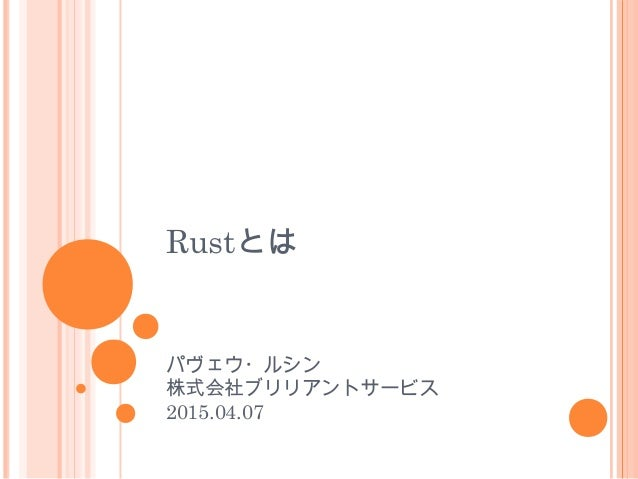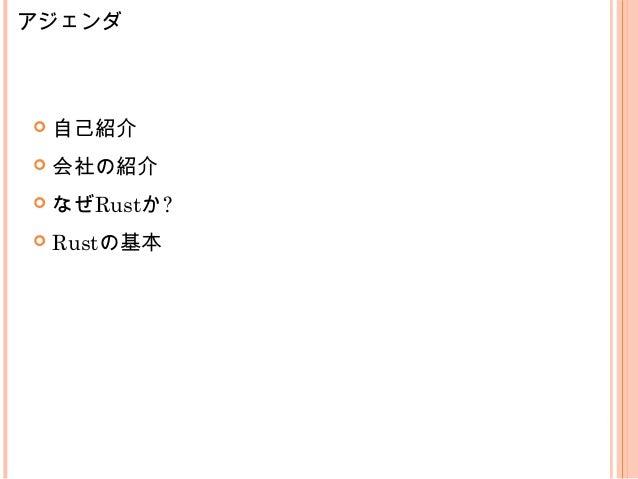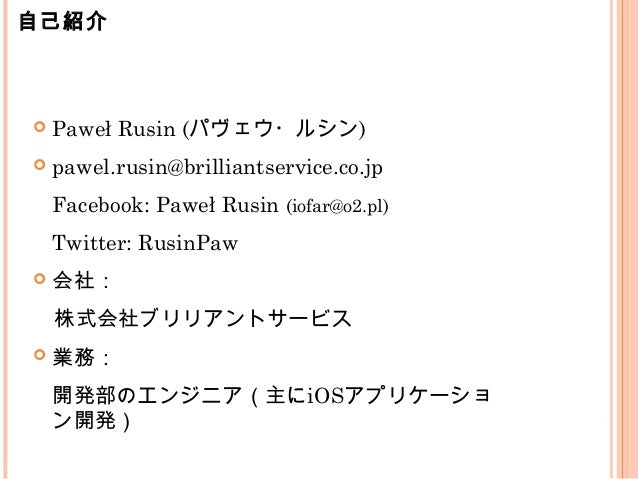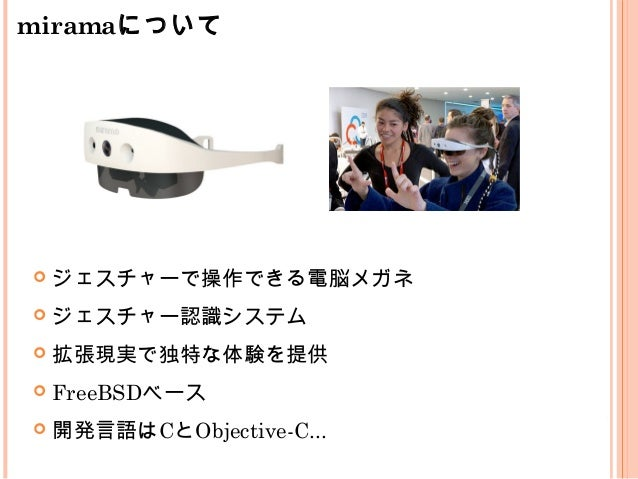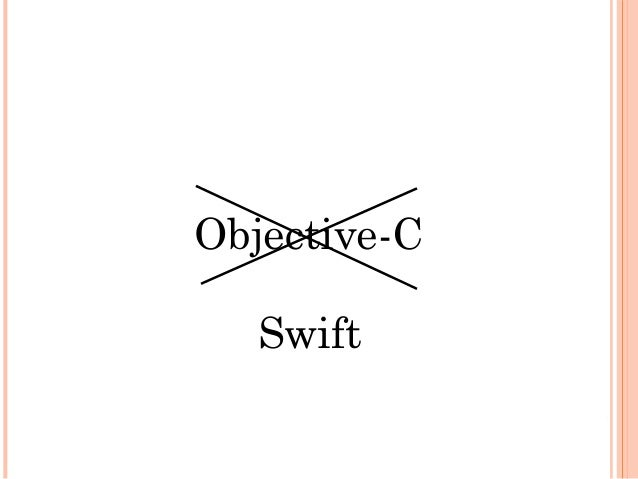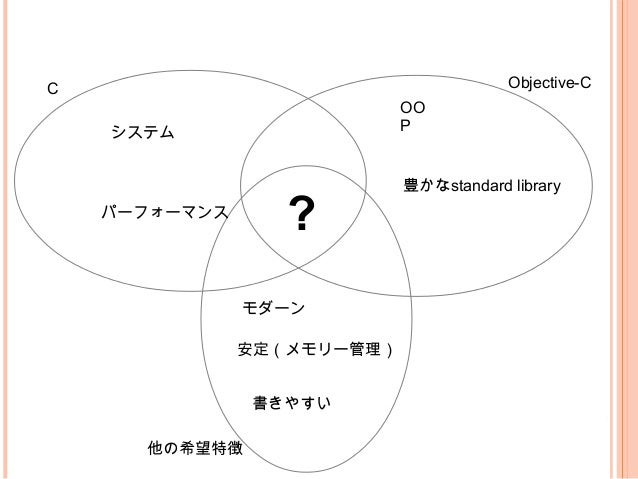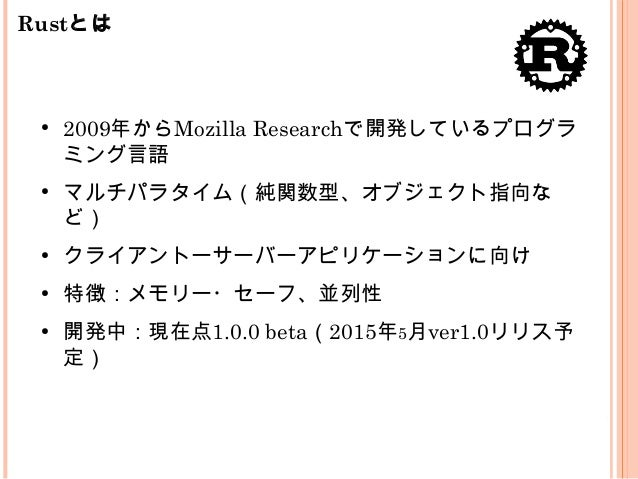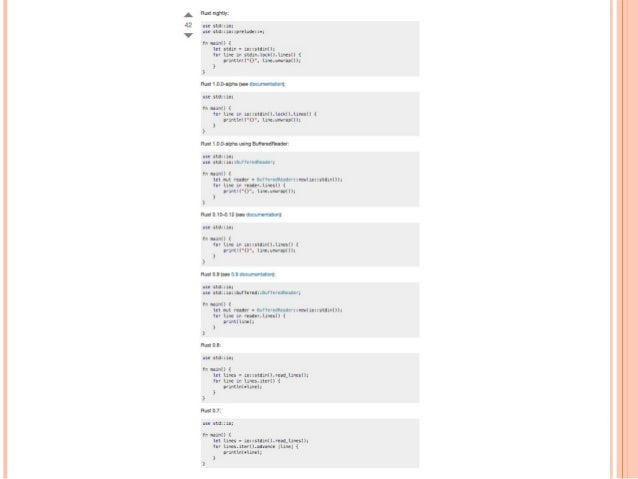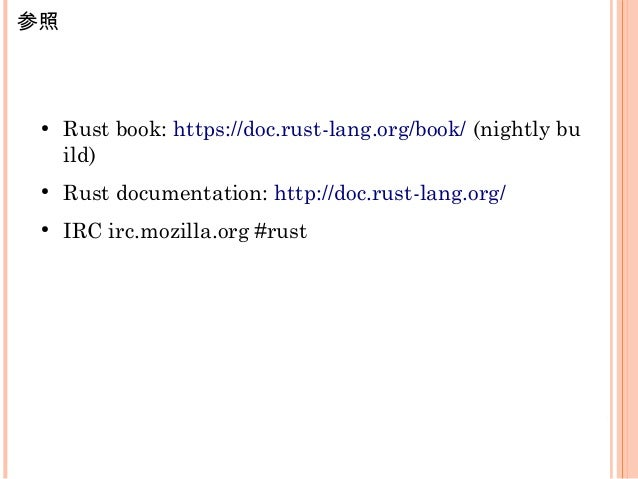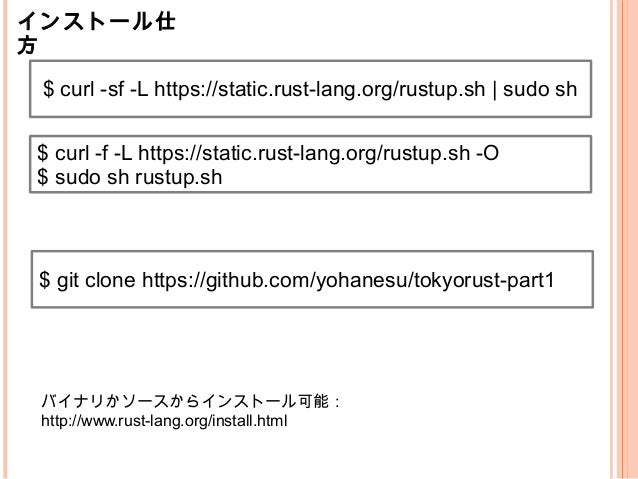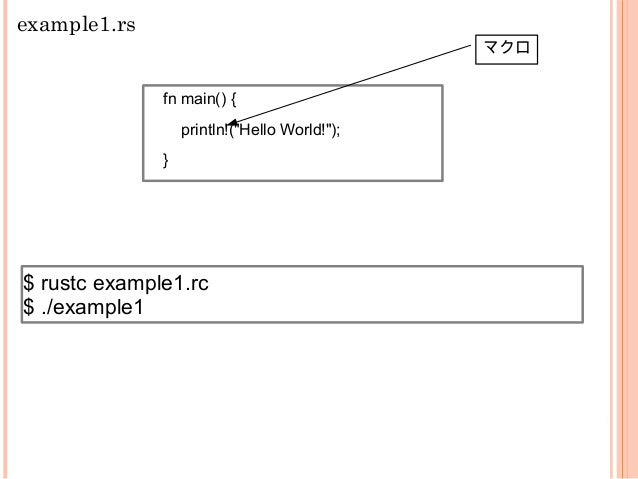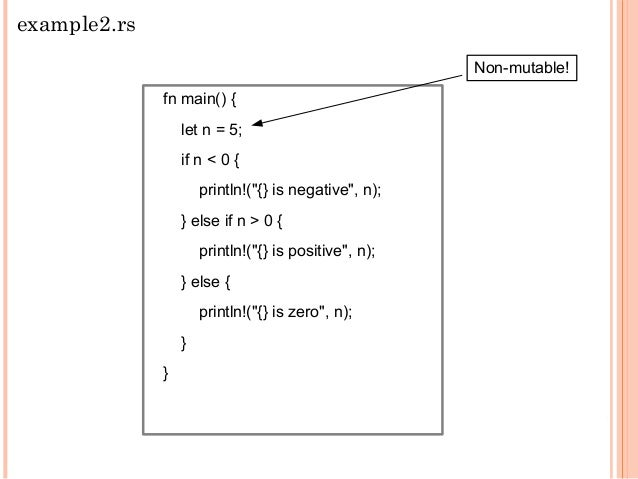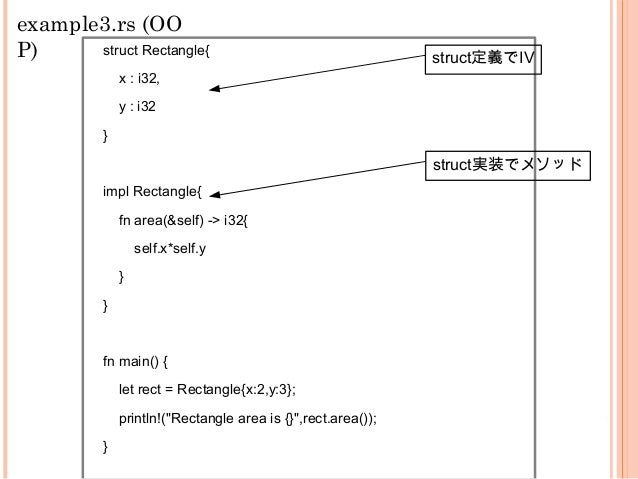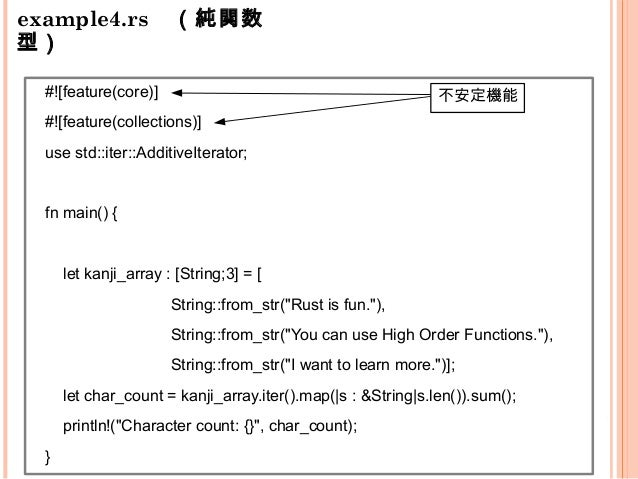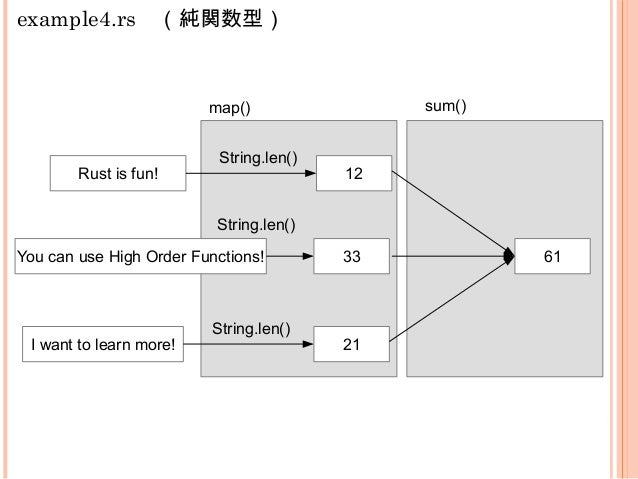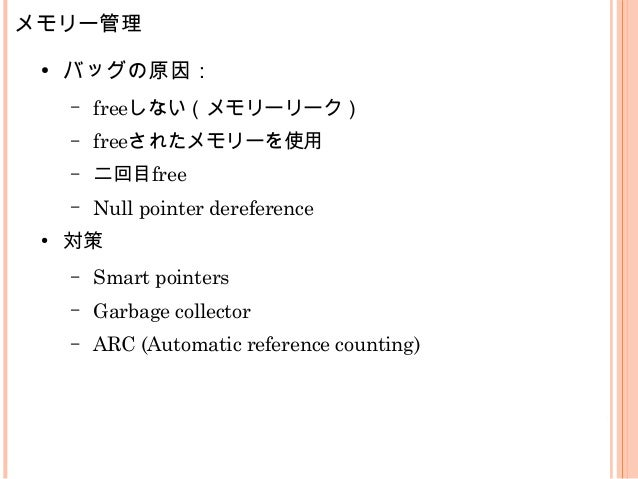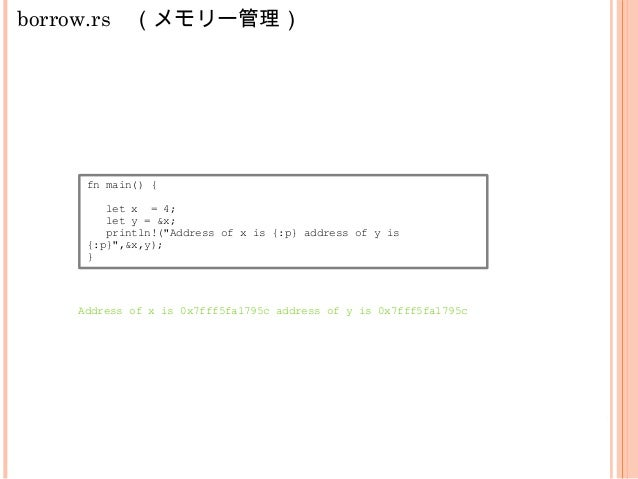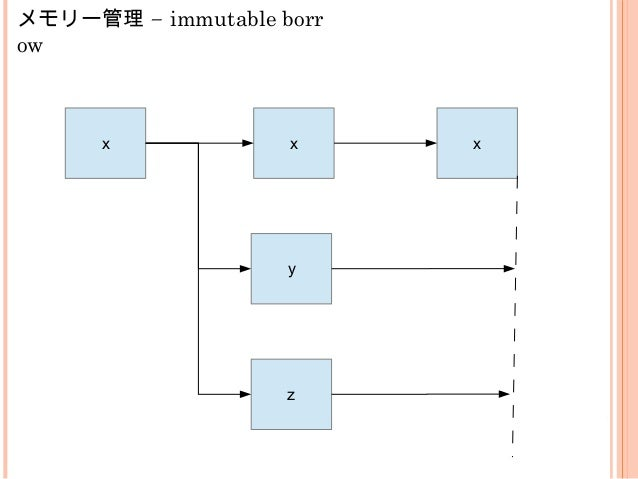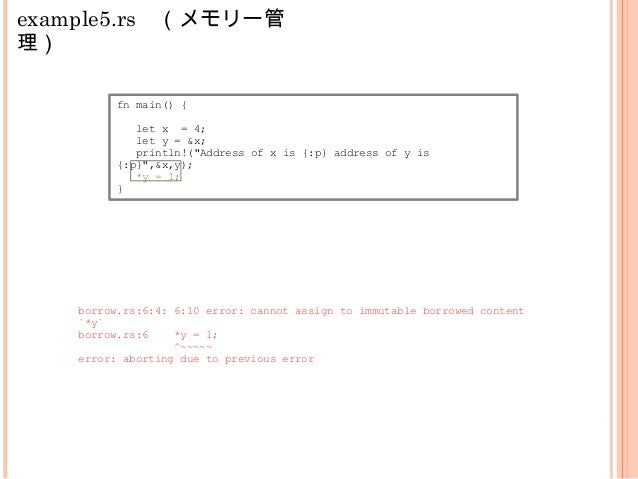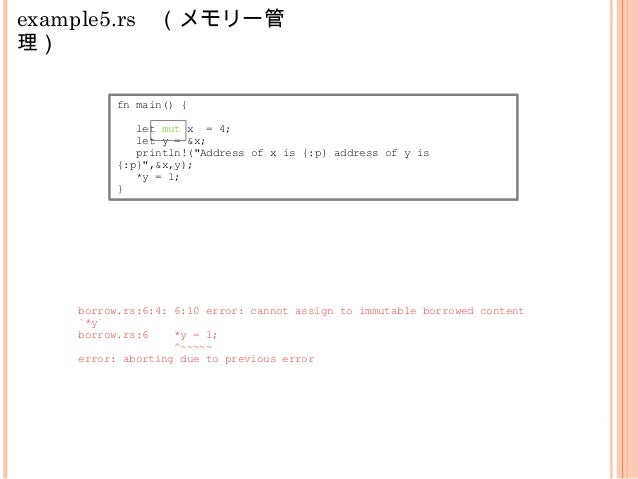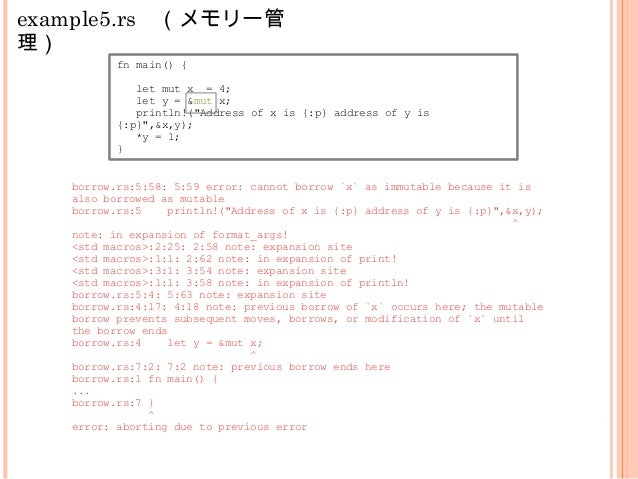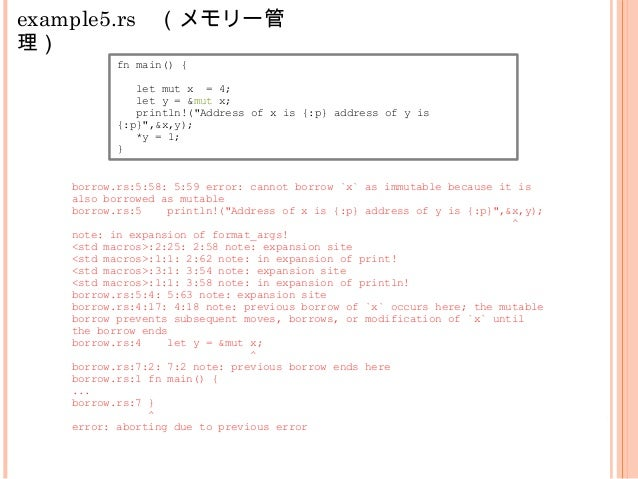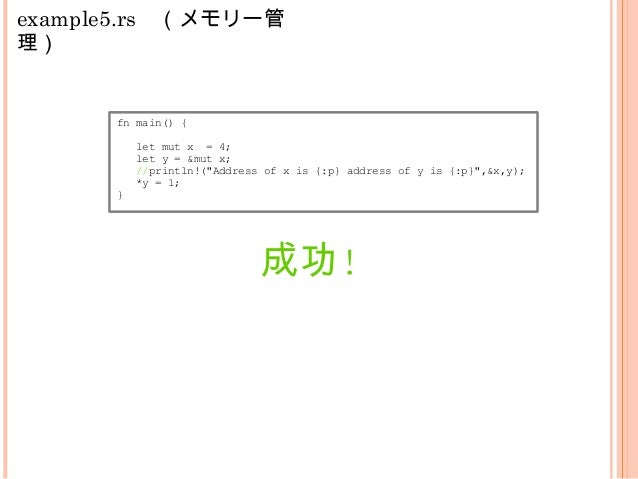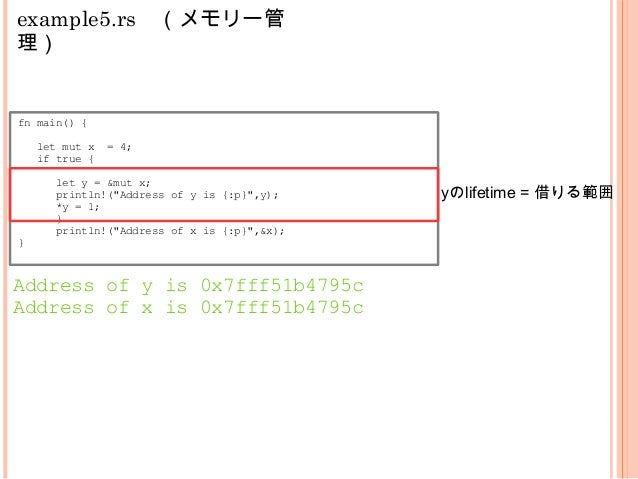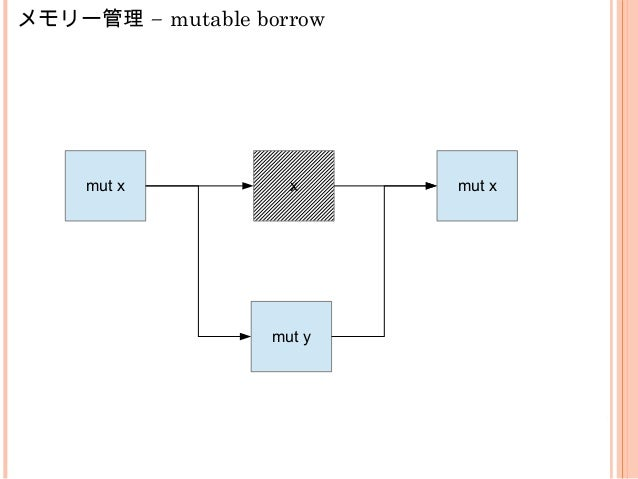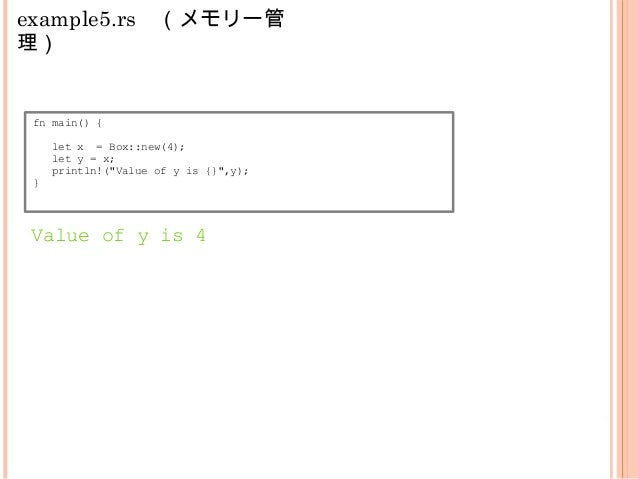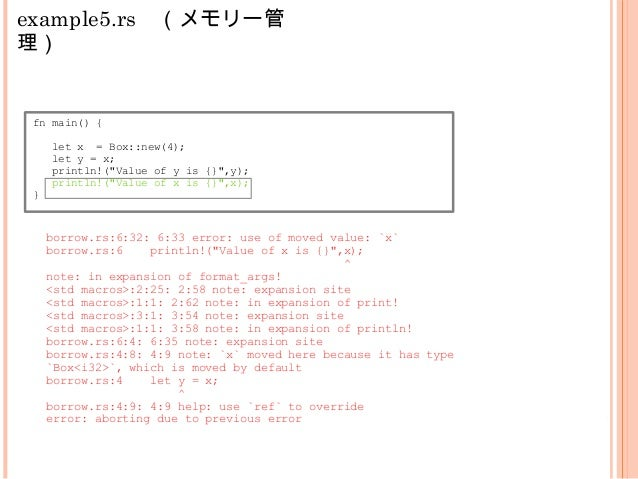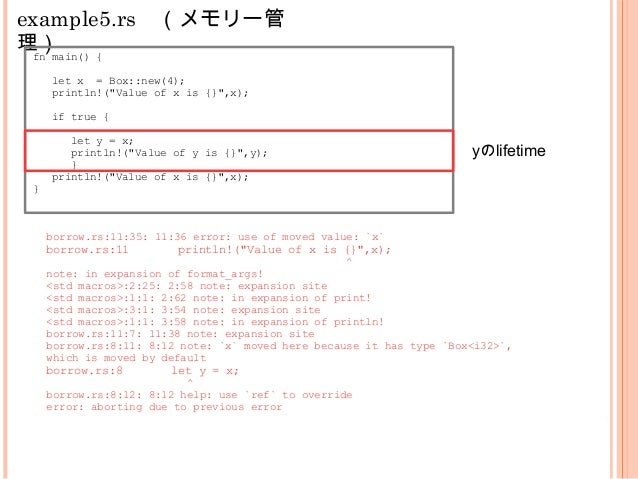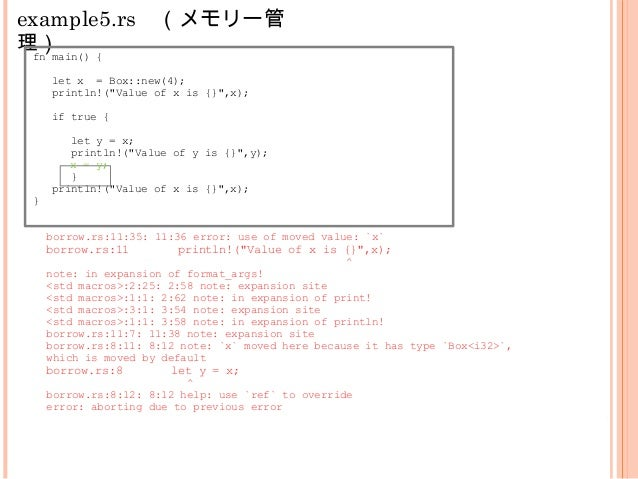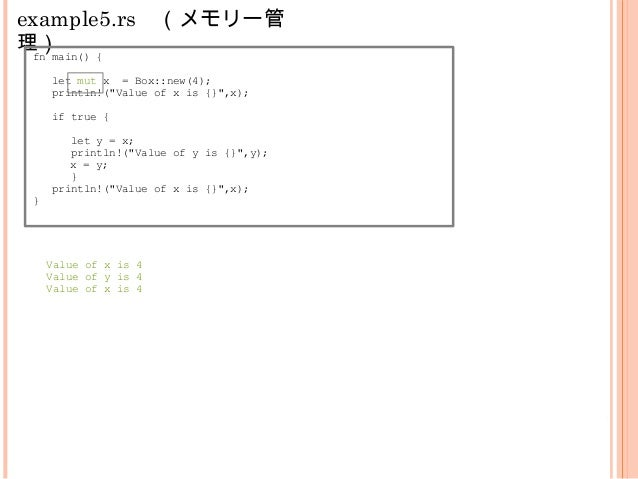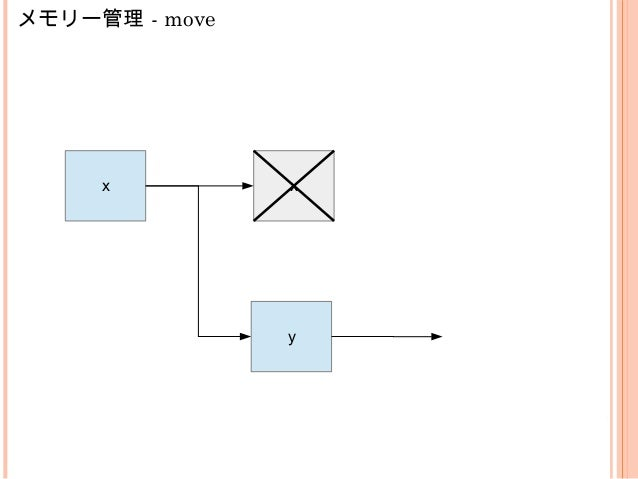Upcoming SlideShare
×
• Full Name
Comment goes here.

Are you sure you want to Yes No• Be the first to comment

### Rust言語紹介

1. 1. Rustとは パヴェウ・ルシン 株式会社ブリリアントサービス 2015.04.07
2. 2.  自己紹介  会社の紹介  なぜRustか?  Rustの基本 アジェンダ
3. 3.  Paweł Rusin (パヴェウ・ルシン)  pawel.rusin@brilliantservice.co.jp Facebook: Paweł Rusin (iofar@o2.pl) Twitter: RusinPaw  会社： 　株式会社ブリリアントサービス  業務： 開発部のエンジニア（主にiOSアプリケーショ ン開発） 自己紹介
4. 4.  株式会社ブリリアントサービス  本社：大阪  支社：東京（品川）、シリコンバレー  アプリケーション開発、O2Oサービス開発、スマートデ バイス開発 会社の紹介
5. 5.  ジェスチャーで操作できる電脳メガネ  ジェスチャー認識システム  拡張現実で独特な体験を提供  FreeBSDベース  開発言語はCとObjective-C... miramaについて
6. 6. Objective-C Swift
7. 7. C Objective-C システム パーフォーマンス OO P 豊かなstandard library ? モダーン 安定（メモリー管理） 書きやすい 他の希望特徴
8. 8. Rustとは ● 2009年からMozilla Researchで開発しているプログラ ミング言語 ● マルチパラタイム（純関数型、オブジェクト指向な ど） ● クライアントーサーバーアピリケーションに向け ● 特徴：メモリー・セーフ、並列性 ● 開発中：現在点1.0.0 beta（2015年5月ver1.0リリス予 定）
9. 9. 参照 ● Rust book: https://doc.rust-lang.org/book/ (nightly bu ild) ● Rust documentation: http://doc.rust-lang.org/ ● IRC irc.mozilla.org #rust
10. 10. \$ curl -sf -L https://static.rust-lang.org/rustup.sh | sudo sh \$ curl -f -L https://static.rust-lang.org/rustup.sh -O \$ sudo sh rustup.sh インストール仕 方 バイナリかソースからインストール可能： http://www.rust-lang.org/install.html \$ git clone https://github.com/yohanesu/tokyorust-part1
11. 11. fn main() { println!("Hello World!"); } example1.rs マクロ \$ rustc example1.rc \$ ./example1
12. 12. fn main() { let n = 5; if n < 0 { println!("{} is negative", n); } else if n > 0 { println!("{} is positive", n); } else { println!("{} is zero", n); } } example2.rs Non-mutable!
13. 13. struct Rectangle{ x : i32, y : i32 } impl Rectangle{ fn area(&self) -> i32{ self.x*self.y } } fn main() { let rect = Rectangle{x:2,y:3}; println!("Rectangle area is {}",rect.area()); } example3.rs (OO P) struct定義でIV struct実装でメソッド
14. 14. example4.rs　（純関数 型） 不安定機能#![feature(core)] #![feature(collections)] use std::iter::AdditiveIterator; fn main() { let kanji_array : [String;3] = [ String::from_str("Rust is fun."), String::from_str("You can use High Order Functions."), String::from_str("I want to learn more.")]; let char_count = kanji_array.iter().map(|s : &String|s.len()).sum(); println!("Character count: {}", char_count); }
15. 15. example4.rs　（純関数型） Rust is fun! You can use High Order Functions! I want to learn more! 12 33 21 61 sum() String.len() String.len() String.len() map()
16. 16. メモリー管理 ● バッグの原因： – freeしない（メモリーリーク） – freeされたメモリーを使用 – 二回目free – Null pointer dereference ● 対策 – Smart pointers – Garbage collector – ARC (Automatic reference counting)
17. 17. borrow.rs　（メモリー管理） fn main() { let x = 4; let y = &x; println!("Address of x is {:p} address of y is {:p}",&x,y); } Address of x is 0x7fff5fa1795c address of y is 0x7fff5fa1795c
18. 18. –メモリー管理 immutable borr ow x x x y z
19. 19. example5.rs　（メモリー管 理） fn main() { let x = 4; let y = &x; println!("Address of x is {:p} address of y is {:p}",&x,y); *y = 1; } borrow.rs:6:4: 6:10 error: cannot assign to immutable borrowed content `*y` borrow.rs:6 *y = 1; ^~~~~~ error: aborting due to previous error
20. 20. example5.rs　（メモリー管 理） fn main() { let mut x = 4; let y = &x; println!("Address of x is {:p} address of y is {:p}",&x,y); *y = 1; } borrow.rs:6:4: 6:10 error: cannot assign to immutable borrowed content `*y` borrow.rs:6 *y = 1; ^~~~~~ error: aborting due to previous error
21. 21. example5.rs　（メモリー管 理） fn main() { let mut x = 4; let y = &mut x; println!("Address of x is {:p} address of y is {:p}",&x,y); *y = 1; } borrow.rs:5:58: 5:59 error: cannot borrow `x` as immutable because it is also borrowed as mutable borrow.rs:5 println!("Address of x is {:p} address of y is {:p}",&x,y); ^ note: in expansion of format_args! <std macros>:2:25: 2:58 note: expansion site <std macros>:1:1: 2:62 note: in expansion of print! <std macros>:3:1: 3:54 note: expansion site <std macros>:1:1: 3:58 note: in expansion of println! borrow.rs:5:4: 5:63 note: expansion site borrow.rs:4:17: 4:18 note: previous borrow of `x` occurs here; the mutable borrow prevents subsequent moves, borrows, or modification of `x` until the borrow ends borrow.rs:4 let y = &mut x; ^ borrow.rs:7:2: 7:2 note: previous borrow ends here borrow.rs:1 fn main() { ... borrow.rs:7 } ^ error: aborting due to previous error
22. 22. example5.rs　（メモリー管 理） fn main() { let mut x = 4; let y = &mut x; println!("Address of x is {:p} address of y is {:p}",&x,y); *y = 1; } borrow.rs:5:58: 5:59 error: cannot borrow `x` as immutable because it is also borrowed as mutable borrow.rs:5 println!("Address of x is {:p} address of y is {:p}",&x,y); ^ note: in expansion of format_args! <std macros>:2:25: 2:58 note: expansion site <std macros>:1:1: 2:62 note: in expansion of print! <std macros>:3:1: 3:54 note: expansion site <std macros>:1:1: 3:58 note: in expansion of println! borrow.rs:5:4: 5:63 note: expansion site borrow.rs:4:17: 4:18 note: previous borrow of `x` occurs here; the mutable borrow prevents subsequent moves, borrows, or modification of `x` until the borrow ends borrow.rs:4 let y = &mut x; ^ borrow.rs:7:2: 7:2 note: previous borrow ends here borrow.rs:1 fn main() { ... borrow.rs:7 } ^ error: aborting due to previous error
23. 23. example5.rs　（メモリー管 理） fn main() { let mut x = 4; let y = &mut x; //println!("Address of x is {:p} address of y is {:p}",&x,y); *y = 1; } 成功!
24. 24. example5.rs　（メモリー管 理） fn main() { let mut x = 4; if true { let y = &mut x; println!("Address of y is {:p}",y); *y = 1; } println!("Address of x is {:p}",&x); } Address of y is 0x7fff51b4795c Address of x is 0x7fff51b4795c yのlifetime = 借りる範囲
25. 25. –メモリー管理 mutable borrow mut x x mut x mut y
26. 26. example5.rs　（メモリー管 理） fn main() { let x = Box::new(4); let y = x; println!("Value of y is {}",y); } Value of y is 4
27. 27. example5.rs　（メモリー管 理） fn main() { let x = Box::new(4); let y = x; println!("Value of y is {}",y); println!("Value of x is {}",x); } borrow.rs:6:32: 6:33 error: use of moved value: `x` borrow.rs:6 println!("Value of x is {}",x); ^ note: in expansion of format_args! <std macros>:2:25: 2:58 note: expansion site <std macros>:1:1: 2:62 note: in expansion of print! <std macros>:3:1: 3:54 note: expansion site <std macros>:1:1: 3:58 note: in expansion of println! borrow.rs:6:4: 6:35 note: expansion site borrow.rs:4:8: 4:9 note: `x` moved here because it has type `Box<i32>`, which is moved by default borrow.rs:4 let y = x; ^ borrow.rs:4:9: 4:9 help: use `ref` to override error: aborting due to previous error
28. 28. example5.rs　（メモリー管 理）fn main() { let x = Box::new(4); println!("Value of x is {}",x); if true { let y = x; println!("Value of y is {}",y); } println!("Value of x is {}",x); } borrow.rs:11:35: 11:36 error: use of moved value: `x` borrow.rs:11 println!("Value of x is {}",x); ^ note: in expansion of format_args! <std macros>:2:25: 2:58 note: expansion site <std macros>:1:1: 2:62 note: in expansion of print! <std macros>:3:1: 3:54 note: expansion site <std macros>:1:1: 3:58 note: in expansion of println! borrow.rs:11:7: 11:38 note: expansion site borrow.rs:8:11: 8:12 note: `x` moved here because it has type `Box<i32>`, which is moved by default borrow.rs:8 let y = x; ^ borrow.rs:8:12: 8:12 help: use `ref` to override error: aborting due to previous error yのlifetime
29. 29. example5.rs　（メモリー管 理）fn main() { let x = Box::new(4); println!("Value of x is {}",x); if true { let y = x; println!("Value of y is {}",y); x = y; } println!("Value of x is {}",x); } borrow.rs:11:35: 11:36 error: use of moved value: `x` borrow.rs:11 println!("Value of x is {}",x); ^ note: in expansion of format_args! <std macros>:2:25: 2:58 note: expansion site <std macros>:1:1: 2:62 note: in expansion of print! <std macros>:3:1: 3:54 note: expansion site <std macros>:1:1: 3:58 note: in expansion of println! borrow.rs:11:7: 11:38 note: expansion site borrow.rs:8:11: 8:12 note: `x` moved here because it has type `Box<i32>`, which is moved by default borrow.rs:8 let y = x; ^ borrow.rs:8:12: 8:12 help: use `ref` to override error: aborting due to previous error
30. 30. example5.rs　（メモリー管 理）fn main() { let mut x = Box::new(4); println!("Value of x is {}",x); if true { let y = x; println!("Value of y is {}",y); x = y; } println!("Value of x is {}",x); } Value of x is 4 Value of y is 4 Value of x is 4
31. 31. メモリー管理 - move x x y
32. 32. 以上 。。。？

### Be the first to comment

•#### junkawa

Apr. 28, 2015
•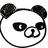#### chikoski

May. 18, 2015

Total views

1,526

On Slideshare

0

From embeds

0

Number of embeds

69

11

Shares

0